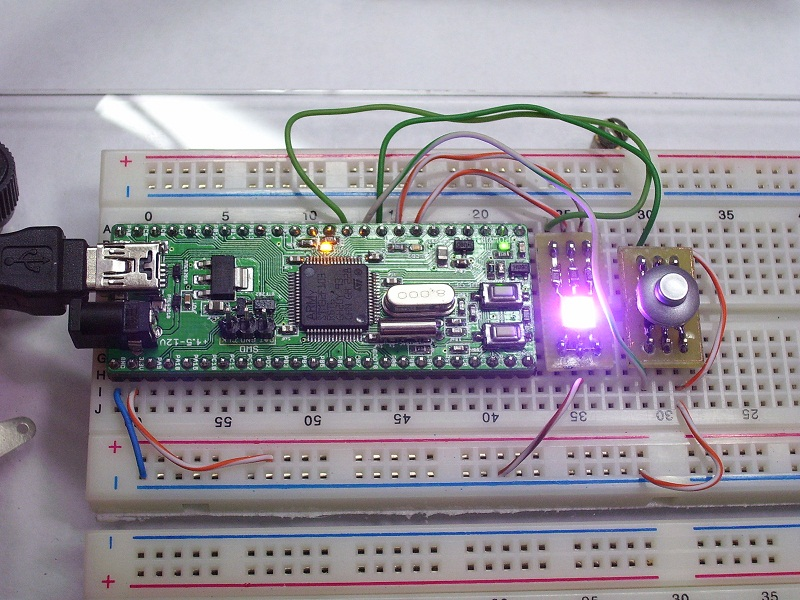## Controlling buttons and RGB LED using Mini64SE R6

Hello everyone, today we see how to to control buttons and RGB LED using STM32 and Mini64SE R6.Open our previous project dedicated to controlling LED using PWM and making the following changes.

```#define PWMR_PORT      GPIOA
#define PWMR_PIN       6
#define PWMR_RCC_BIT   RCC_APB2Periph_GPIOA

#define PWMG_PORT      GPIOA
#define PWMG_PIN       7
#define PWMG_RCC_BIT   RCC_APB2Periph_GPIOA

#define PWMB_PORT      GPIOB
#define PWMB_PIN       0
#define PWMB_RCC_BIT   RCC_APB2Periph_GPIOB

#define BUT_PORT      GPIOC
#define BUT1_PIN       4
#define BUT2_PIN       5
#define BUT_RCC_BIT   RCC_APB2Periph_GPIOC

unsigned char But1Val=0;
unsigned char But2Val=0;```

Here we add an adjustment for the  RGB LED control pin PA6, PA7, PB0 and inputs pins for two buttons PC4, PC5. As Buttons I use joystick from mobile phone. We will ask joystick pressing up or downhill.
We also see your two variables are assigning to the survey mechanism antirattle for buttons.

```GPIO_InitStructure.GPIO_Pin = (1 << PWMR_PIN);
GPIO_InitStructure.GPIO_Speed = GPIO_Speed_2MHz;
GPIO_InitStructure.GPIO_Mode = GPIO_Mode_AF_PP;
GPIO_Init(PWMR_PORT, &GPIO_InitStructure);

GPIO_InitStructure.GPIO_Pin = (1 << PWMG_PIN);
GPIO_InitStructure.GPIO_Speed = GPIO_Speed_2MHz;
GPIO_InitStructure.GPIO_Mode = GPIO_Mode_AF_PP;
GPIO_Init(PWMG_PORT, &GPIO_InitStructure);

GPIO_InitStructure.GPIO_Pin = (1 << PWMB_PIN);
GPIO_InitStructure.GPIO_Speed = GPIO_Speed_2MHz;
GPIO_InitStructure.GPIO_Mode = GPIO_Mode_AF_PP;
GPIO_Init(PWMB_PORT, &GPIO_InitStructure);

GPIO_InitStructure.GPIO_Pin = (1 << BUT1_PIN)|(1 << BUT2_PIN);
GPIO_InitStructure.GPIO_Speed = GPIO_Speed_2MHz;
GPIO_InitStructure.GPIO_Mode = GPIO_Mode_IPU;
GPIO_Init(BUT_PORT, &GPIO_InitStructure);```

Setting  pins on the board Mini64SE R6 for input or output according to the configuration described above.

```TIM3->CCER |= TIM_CCER_CC1E|TIM_CCER_CC2E|TIM_CCER_CC3E|TIM_CCER_CC4E; //Allow timer use CH4 pin
TIM3->CCMR1|=( TIM_CCMR1_OC1M_0|TIM_CCMR1_OC1M_1|TIM_CCMR1_OC1M_2|TIM_CCMR1_OC2M_0|TIM_CCMR1_OC2M_1|TIM_CCMR1_OC2M_2);//Select inverce PWM on CH1 CH2 pin
TIM3->CCMR2|=( TIM_CCMR2_OC3M_0|TIM_CCMR2_OC3M_1|TIM_CCMR2_OC3M_2|TIM_CCMR2_OC4M_0|TIM_CCMR2_OC4M_1|TIM_CCMR2_OC4M_2);//Select inverce PWM on CH3 CH4 pin
TIM3->CR1 |= TIM_CR1_CEN;// Starting timer```

Setting  the timer and its channels CH1, CH2, CH3 to control RGB LED via PWM.

Let’s move on to the main code.

```  TIM3->CCR1=pwm_arr;
TIM3->CCR2=pwm_arr;
TIM3->CCR3=pwm_arr;
TIM3->CCR2=pwm_arr;//Set the LEDS mode of the minimum brightness
srand(time(0));
while (1)
{

{

But1Val++;
Delay(4);
if (But1Val==250)
{
//Tics+=10;
But1Val=0;
TIM3->CCR1=pwm_arr;//If you press the first button LEDs are OFF
TIM3->CCR2=pwm_arr;
TIM3->CCR3=pwm_arr;
TIM3->CCR4=pwm_arr;
}
}
else
{
But1Val=0;
}

{

But2Val++;
Delay(4);
if (But2Val==250)
{
//Tics+=10;
But2Val=0;
TIM3->CCR1=rand();//If you press the second button we load in the PWM registers random values ​​of brightness
TIM3->CCR2=rand();
TIM3->CCR3=rand();
TIM3->CCR4=rand();